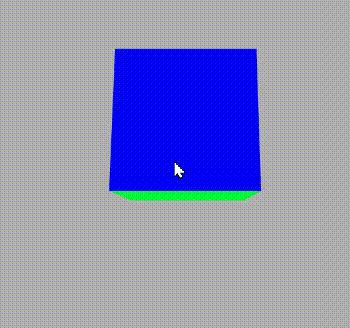# p5.js exitPointerLock() Function

• Last Updated : 01 Apr, 2021

The function exitPointerLock() quit the previously used Pointer Lock for example if the pointer is locked then it unlock it and vice-versa. It is used to exit the pointer lock  which is called by requestPointer() function.

Syntax:

`exitPointerLock()`

Step 1: Open the web editor of p5.js https://editor.p5js.org/

Step 2:Write the following code and see the result.

Example:

## Javascript

 `// Make a boolean variable and set ` `// its value false ` `let lock = ``false``; ` ` `  `// Set the function ` `function` `setup() { ` ` `  `    ``// Set the frame rate ` `    ``frameRate(5); ` ` `  `    ``// Set the canvas size ` `    ``createCanvas(600, 400, WEBGL); ` `} ` ` `  `// Set the draw function ` `function` `draw() { ` ` `  `    ``// Set the background colour ` `    ``background(175); ` ` `  `    ``// Set the camera ` `    ``let cX = random(-10, 10); ` `    ``let cY = random(-10, 10); ` `    ``let cZ = random(-10, 10); ` ` `  `    ``camera(cX, cY, ` `        ``cZ + (height / 2) / tan(PI / 6), ` `        ``cX, 0, 0, 0, 1, 0); ` ` `  `    ``ambientLight(255); ` ` `  `    ``rotateX(-movedX * 0.1); ` `    ``rotateY(movedY * 0.1); ` ` `  `    ``noStroke(); ` `    ``normalMaterial(); ` ` `  `    ``box(100, 100, 100); ` `} ` ` `  `// Function to apply exitpointer lock ` `function` `mouseReleased() { ` ` `  `    ``// If lock is not false then ` `    ``// make it true ` `    ``if` `(!lock) { ` `        ``lock = ``true``; ` ` `  `        ``// Request for pointer lock ` `        ``requestPointerLock(); ` `    ``} ``else` `{ ` ` `  `        ``// Exit the pointer lock ` `        ``exitPointerLock(); ` ` `  `        ``// Again make te lock variable false ` `        ``lock = ``false``; ` `    ``} ` `} `

Output:In output, we can see that when the mouse is released it exit the previous pointer lock because we have set the mouseReleased() function to exit the pointer lock.

My Personal Notes arrow_drop_up
Recommended Articles
Page :